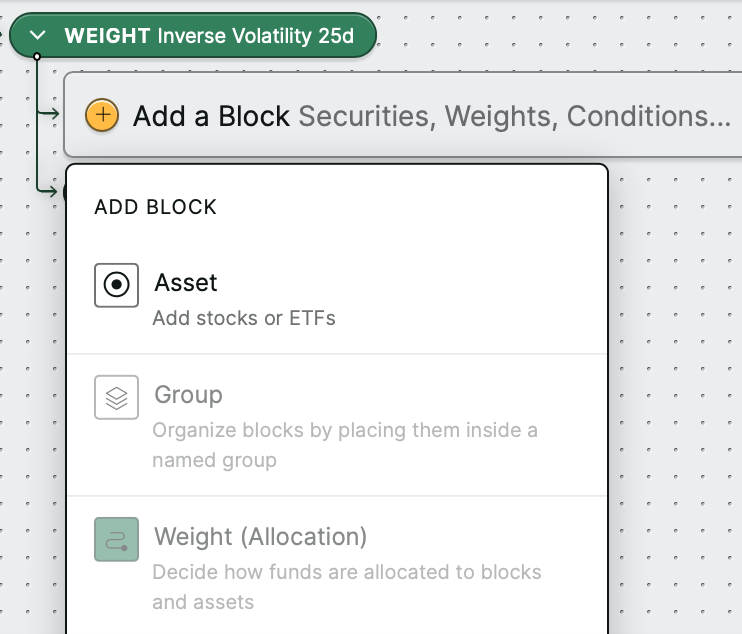Inverse volatility weighting

In this article, you'll learn how inverse volatility weighting works and when it can be used.

How does inverse volatility weighting work?

With inverse volatility weighting, assets are weighted in inverse proportion to the volatility of their returns. Assets that are more volatile receive lower weights. Selecting a lookback period is required to calculate assets' volatility.

Volatility is measured by the standard deviation (SD) of the percent returns of each asset over the selected lookback period. An asset's inverse volatility is 1/SD of the percent returns. The share of weight that each asset receives is equal to its inverse volatility divided by the total inverse volatility of all assets.

When can inverse volatility weighting be used?

This kind of weighting can only be used with assets (stocks or ETFs), and not other kinds of blocks. If you create a new inverse volatility weight, you will only be able to add assets under it.